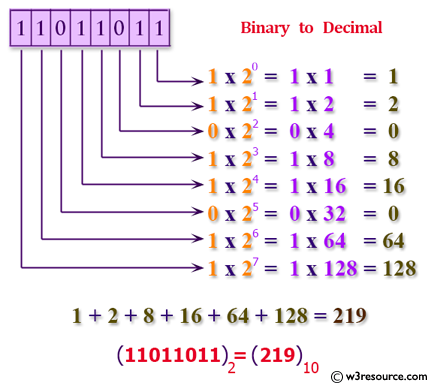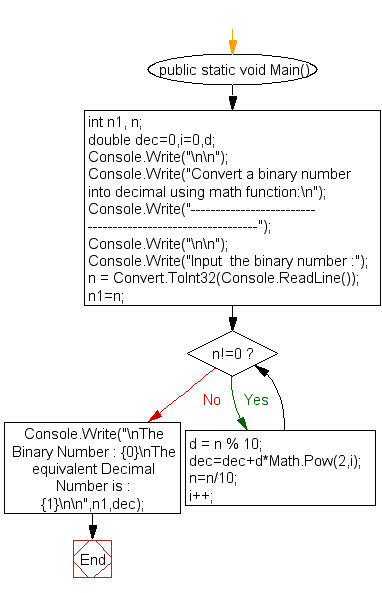﻿ C# - Binary number to a decimal using math function# C# Sharp Exercises: Convert a binary number into a decimal using math function

## C# Sharp For Loop: Exercise-46 with Solution

Write a program in C# Sharp to convert a binary number into a decimal number using math function.

Pictorial Presentation:Sample Solution:

C# Sharp Code:

``````using System;
public class Exercise46
{
public static void Main()
{   int n1, n;
double dec=0,i=0,d;

Console.Write("\n\n");
Console.Write("Convert a binary number into decimal using math function:\n");
Console.Write("-----------------------------------------------------------");
Console.Write("\n\n");

Console.Write("Input  the binary number :");
n1=n;
while(n!=0)
{  d = n % 10;
dec=dec+d*Math.Pow(2,i);
n=n/10;
i++;
}
Console.Write("\nThe Binary Number : {0}\nThe equivalent Decimal  Number is : {1}\n\n",n1,dec);
}
}
```
```

Sample Output:

```Convert a binary number into decimal using math function:
-----------------------------------------------------------
Input  the binary number :1000001
The Binary Number : 1000001
The equivalent Decimal  Number is : 65
```

Flowchart:C# Sharp Code Editor:

Contribute your code and comments through Disqus.

What is the difficulty level of this exercise?

Test your Programming skills with w3resource's quiz.

﻿